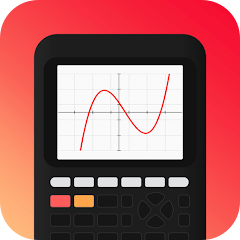# Taculator Graphing Calculator

3.7
343 reviews
10K+Everyone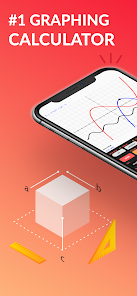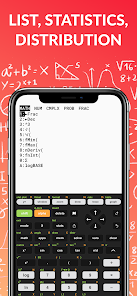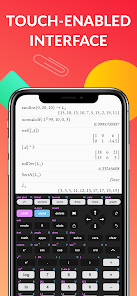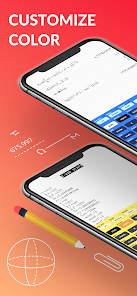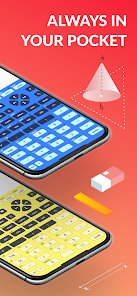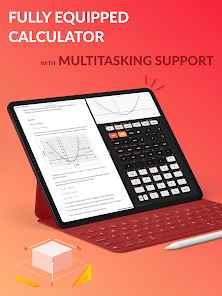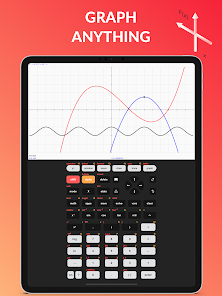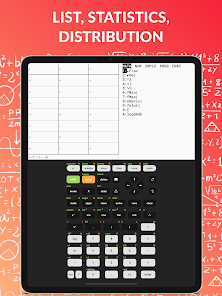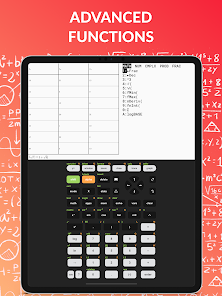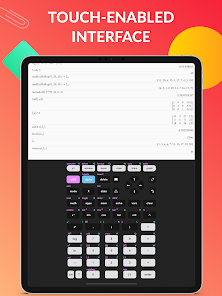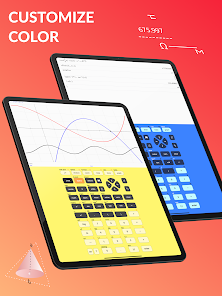TACULATOR GRAPHING CALCULATOR
• Your graphing calculator for high school and college students.
• Includes all the commands and functions for advanced math, list, statistics, distribution, stat plots, etc.
• Use the arrow keys or your fingers to navigate inside the app.
• Covers everything you need: Pre-Algebra, Algebra 1 & 2, Pre-Calculus, Calculus, Statistics...

IT'S EVEN BETTER THAN AN ACTUAL CALCULATOR
• Taculator is 100X faster as it uses the huge calculation power of your iPhone/iPad, while handheld calculators like TI-84 or Casio are often slow.
• High-resolution graphing window: Make sharp and beautiful graphs.
• Share your calculations with friends as a text message or email.

◉ Math Functions
• Scientific calculator with all basic arithmetic and advanced functions.
• Ability to scroll through history and copy previous calculations.
• Complex numbers support
• Probability functions like nPr, nCr, factorial, random numbers, 84.
• Enter fractions easily.
• Convert angles and use DMS format.

◉ Graphing Functions
• Graph every function you want and trace for X- and Y-values
• Calculate intersection, zero, minimum, maximum, integral, ti, and derivative.
• Switch between full-screen graphs and normal mode.
• Zoom in and out with your fingers and adjust the window settings.
• Graph statistical plots like histogram, box plot, scatter plot, xy-line plot
• Draw vertical, horizontal, tangent, circle, ti.

◉ Matrix Calculator 84
• Store matrices and reuse them using shortcuts like [A], [B], [C],...
• Do matrix arithmetic operations: Scalar multiplication, addition & subtraction, multiplication, power of a matrix.
• Calculate transpose, determinant, dimension, inverse matrix, row echelon form, reduced row echelon form, identity matrix, cumulated sum.

◉ List, Statistics, and Distribution
• Use the stat list editor to enter your list values.
• Perform 1- and 2-Variable statistics.
• Use lists to calculate min, max, mean, median, sum, product, standard deviation, variance.
• Find best-fit regressions: LinReg (ax+b), LinReg (a+bx), QuadReg, CubicReg, QuartReg, LnReg, ExpReg, PwrReg, Logistic, SinReg.
• Perform statistical tests: Z-Test, T-Test, 2-SampleZTest, 2-SampleTTest, 1-PropZTest, 2-PropZTest, TI calculator, ZInterval, TInterval, 2-SampleZInterval, 2-SampleTInterval, 1PropZInterval.
• Calculate various distributions: Normalpdf/cdf, invT, Tpdf/cdf, χ2pdf/cdf, Fpdf/cdf, Poissonpdf/cdf 84, Binompdf/cdf, Geometpdf/cdf.

◉ Command Catalog & Syntax Help
• Get help with all commands with our integrated Catalog & Help section.
• It provides you with syntax help, examples, and possible error messages.
• Enter a command and the app will show you the right syntax next to the cursor.

SUPPORT

---
For extended features such as more advanced calculations and functions, get the Taculator Pro membership.

https://www.taculator.com/terms-of-service.html

https://www.taculator.com/privacy-policy.html
Updated on
Jan 5, 2022

## Data safety

Safety starts with understanding how developers collect and share your data. Data privacy and security practices may vary based on your use, region, and age. The developer provided this information and may update it over time.No data shared with third partiesNo data collected
3.7
343 reviews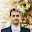Sander Lesage
May 26, 2022
I like it! Vibrate on keypress would be a nice addition. I also have some small issues where selecting a previous result shows an empty pop menu when in darkmode (switching darkmode also shows the let's get started menu again). The up arrow also does not select the previous result.
3 people found this review helpful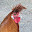Eric Kohler
September 11, 2021
Graphing doesn't work. I entered 3 equations, hit the graph button, and after a 3 second delay, a blank graph appears. I tried very simple equations (y = 2x, y = -x, etc), made sure the minimum and maximum axis values were appropriate (-10 to 10 on both axes), and it won't plot the graphs. I'll try a different app.
15 people found this review helpful
Calculator & Geometry Apps
September 16, 2021
Hi, we aren't able to reproduce this error. Did you do other calculates before the graphing stopped working?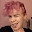Jessi Ellis
June 4, 2022
I'm pretty frustrated that the functions you can easily get for free on your standard phone calculator are the only free functions on here. You're better off spending your money on an actual graphing or stats calculator
8 people found this review helpful